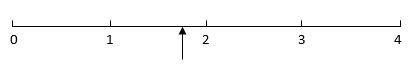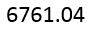# Estimation and Place Value

## Estimation

For example:

Estimate the answer to this sum: 4.09 x 6.98

Of course, you could work it out. It'd waste a bit of time and wouldn't get any extra marks at all. The trick is to say '4.09 is almost the same as 4, 6.98 is almost the same as 7'. Given that one is slightly over the estimate and the other slightly under, the answer of 28 (4 x 7) is really likely to be accurate.

Other estimation questions have no definite answer and tend to be about reading scales, graphs or estimating position on a number line. In instances where you need to estimate as the actual answer isn't clear-cut, precision is a good thing. Look at this example:

Estimate what figure is the arrow pointing to:In a question like this, whereby a definite answer is impossible, encourage your child to think about the answer they have put and see whether it is actually impossible. For example, if they have a ruler they should be able to measure how far it is between each number and therefore get a more accurate idea of whether the arrow is pointing to 1.75, 1.7 or 1.8. If they were to say 1.6, or 1.9, the ruler would help show that it was incorrect. If they said 1.9, it would mean the gap between the arrow and the number 2 was nine times smaller than the distance from the arrow and the number 1. It clearly isn't!

There is usually a small margin of error allowed in estimation questions and in this one, I'd accept anything from 1.7 to 1.8 inclusive.

## Place Value

One of the foundations of maths is the concept of place value. Ever since we adopted the base ten Hindu-Arabic number system, life for mathematicians became more understandable. Rather than the alternatives, such as Roman numerals, hexadecimal or binary, this system allows ease of calculation on paper. The positioning of digits in the correct 'column' and with the decimal point in the right place is fundamental to its success.

Children will get tested on their understanding in different ways and have to be able to understand the question in order to know what is expected of them. I always feel such questions are very easy but are not fair as they can be interpreted differently according to the structure of the question.

Look at the following number:What does the 7 represent?

The answer is 700. The 7 is in the hundreds column so it shows us that there are seven hundreds in this number.

However, a similar question could ask which column the 7 is in. In this case it would be in the hundreds - that would be the answer rather than 700. Like I say, the understanding is usually there but the question is awkward!

If in doubt yourself, look at the following order of place value in a number.

 Millions Hundred Thousands Ten Thousands Thousands Hundreds Tens Ones . Tenths Hundredths 1 6 4 2 9 8 5 . 3 7

The number as it is normally written is

1 642 985.37

Your child would be expected to state that the value of the '2' in that number was '2000' or that the value of the '3' was '0.3'